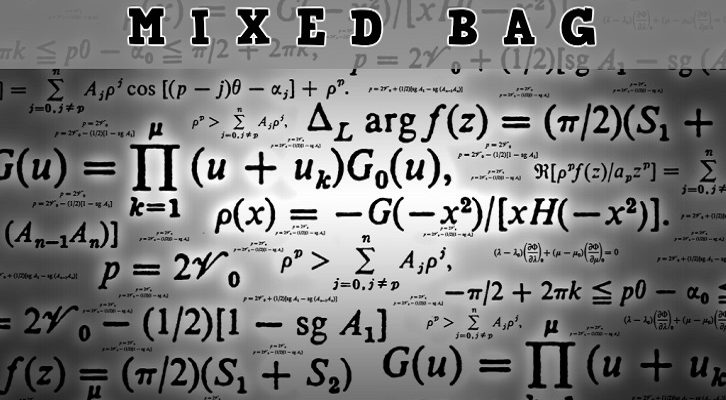# CAT Functions – Questions

Have given below a few questions on functions.

1. Give the domain and range of the following functions

i. f(x) = x2 + 1

ii. g(x) = log (x + 1)
iii. h(x) = 2x
iv. f(x) = 1/ (x+1)
v. p(x) = | x + 1|
vi. q(x) = [2x], where [x] gives the greatest integer less than or equal to x

Happy cracking